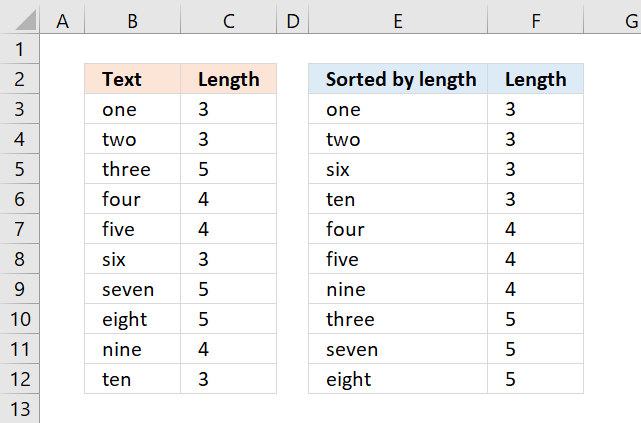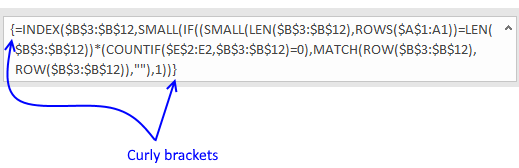Author: Oscar Cronquist Article last updated on December 14, 2017The array formula in column E, shown in above picture sorts text values from column B. The Length columns prove that they are sorted by text length from small to large.

=INDEX(\$B\$3:\$B\$12, SMALL(IF((SMALL(LEN(\$B\$3:\$B\$12), ROWS(\$A\$1:A1))=LEN(\$B\$3:\$B\$12))*(COUNTIF(\$E\$2:E2, \$B\$3:\$B\$12)=0), MATCH(ROW(\$B\$3:\$B\$12), ROW(\$B\$3:\$B\$12)), ""), 1))

To enter an array formula, type the formula in a cell then press and hold CTRL + SHIFT simultaneously, now press Enter once. Release all keys.The formula bar now shows the formula with a beginning and ending curly bracket telling you that you entered the formula successfully. Don't enter the curly brackets yourself.

If you are looking for a formula that sorts text alphabetically, check this out: Sort a column alphabetically